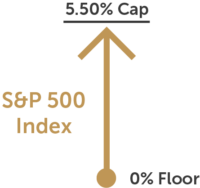Annuities come in a variety of ways. Some are very useful tools for investors seeking safety of their principal. Others are high cost rip-offs meant to pay your broker large commissions and lock the investor into a poor investment product. At FMF365.com we ONLY consider high quality, no cost fixed annuities, as a portion of your overall investment portfolio.

Fixed annuities have evolved into two basic rate paying methods:

Declared Rate Fixed Annuities

% Rate of interest is stated and guaranteed for a specific period of time

Indexed Fixed Annuities

Fixed rates just like plain fixed annuities. Plus the option to have your interest credit tied to the performance of a stock index such as the S&P 500

Let’s look at how indexed fixed annuities work and the key terms you’ll want to know when researching about them.

Indexing Method:

The indexing method means the approach used to measure the amount of change, if any, in the index. Some of the most common indexing methods include averaging, high-water mark, and point-to-point.

Term:

The index term is the period during which index-linked interest is calculated; the interest is credited to your annuity at the end of the term. Terms are generally from one to 10 years, with six or seven years being most common. Some annuities offer single terms while others offer multiple, consecutive terms. If an annuity has multiple terms, there will usually be a window at the end of each term (typically 30 days) when you may withdraw your money witout penalty. For installment premium annuities, the payment of each premium may begin a new term for that premium.

Participation Rate:

The participation rate determines how much of the increase in the index will be used to calculate index-linked interest. For example, if the calculated change in the index is 9% and the participation rate is 70%, the index-linked interest rate will be 6.3% (9% x 70% = 6.3%). A company may change the participation rate for newly issued annuities as often as each day. Therefore, the initial participation rate is for a specific period (from one year to the entire term). When that period is over, the company sets a new participation rate for the next period. Some annuities guarantee that the participation rate will never be set lower than a specified minimum or higher than a specified maximum. Not all annuities have a participation rate that fluctuates.

Cap Rate:

Some annuities may place an upper limit, or cap, on the index-linked interest rate. This is the maximum rate of interest the annuity will earn. In the example above, if the contract has a 6% cap rate, 6%, not 6.3%, would be credited. Not all annuities have a cap rate.

The floor is the minimum index-linked interest rate that can be credited. The most common floor is 0%. A 0% floor assures even if the index decreases, in value, the index-linked interest credited will be zero and not negative. As in the case of a cap, not all annuities have stated floor or index-linked interest rates. But in all cases, fixed annuities will have a minimum guaranteed value calculated separately from the index-linked value.

Averaging:

In some annuities, the average of the index’s value is used rather than the actual value of the index on a specified date. The index averaging may occur at the beginning, the end, or throughtou the entire term of the annuity.

Interest Compounding:

Some annuities pay simple interest during an index term, which means index-linked interest is added to the original premium amount, but does not compound during the term. Others pay compound interest during a term, which means that index-linked interst that has already been credited also earns interest in the future.

In some annuities, the index-linked interest rate is computed by subtracting a specific percentge from any calculated change in the index. This percentage, sometimes referred to as the “margin” or “spread” might be used instead of, or in addition to, a participation rate. For example, if the calculated change in the index is 10%, the annuity might specify that 2.25% will be subtracted from the rate to determine the interest rate credited. Here, the rate would be 7.75% (10% – 2.23% = 7.75%). In this example, the company subtracts the percentage only if the change in the index produces a positive interest rate.

Vesting:

Some annuities credit none of the index-linked interest, or only part of it, if money is removed before the end of the term. The percentage that is vested, or credited, generally increases as the term comes close to its end and is always 100% at the end of the term.

Now that you know all the terms and important parts to any indexed fixed annuity, its important to know there’s several factors that will alter how current rates for all these different investment buckets you can invest in. Interest rates, insurance company quality, market volatility all are factors that will make rates change on new product offerings.

Here’s an example of how an Indexed Annuity might look:

Indexed Fixed Annuity

\$100,000 Investment

Locked in for a period of time, such as 5, 7, or 10 years.

Partial Liquidity every year usually 10% of initial investment each year (\$10,000 per year in this example)

Investment can be broken up into different buckets:

Bucket #1: Declared Rate

# 2.00%(example)

Bucket #2: Cap Rate Index1 Year

Bucket #3: Participation Rate1 Year